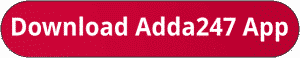Latest Banking jobs   »   bank exam 2023

# Quantitative Aptitude Quiz For Bank Mains Exam 2023-29th October

Directions (1-4): Pie-chart shows the distribution of students in 5 different streams of a college. And table given below shows the passing % of boys, girls and total students within the streams. Study the data carefully and solve the following questionsNote:
(i) There are only 5 streams are in college.
(ii) Some values are missing you have to calculate it according to questions.

Q1. Number of girls in stream E is 200% more than the number of boys in same stream. What is the passing percentage of the girls in stream E. (approx.)
(a) 84%
(b) 87%
(c) 92%
(d) 62%
(e) 70%

Q2. If ratio between number of boys to number of girls in stream A is 2 : 3 then find the total passing percentage of students in stream A.
(a) 48%
(b) 44%
(c) 46%
(d) 54%
(e) 50%

Q3. Number of boys passed in stream E is 76 more than that of stream B and total student passed in stream B is 252 more than that of stream E. Find the % of girls passed in stream E.
(a) 72%
(b) 82%
(c) 98%
(d) 92%
(e) 86%Q5.If we place (X) at the left end of a five-digit number we will get a six-digit number which is four-times the six-digit number that we get when we put (X) at the right end of the original five-digit number. Then which of the following will be the pair of X and the sum of digits of the original five digits number?
I. 9, 18 II. 5, 13 III. 7, 23 IV. 6, 18 V. 8, 25
(a) I, II and IV
(b) I, III and V
(c) All of the above
(d) Both I and II
(e) II, III and IV

Solutions.                                                                                       .

## FAQs

### When is the IBPS Clerk Mains exam scheduled for?

The IBPS Clerk Mains exam is scheduled for 7 October 2023.

#### Congratulations!Union Budget 2023-24: Free PDF